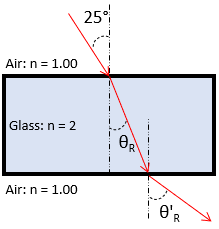## Snell’s Law

Consider sunlight travelling through the air strikes a flat slab of glass at an incident angle of 25°. If the glass’s index of refraction is 2, what is the light’s angle of refraction at the moment it leaves the glass? Note the index of refraction for air is 1.

HintHint 2
Snell’s Law:
$$n_isin\theta_i=n_rsin\theta_r$$$where $$n_i$$ is the incident index, $$n_r$$ is the refracted index, $$\theta_i$$ is the incident angle, and $$\theta_r$$ is the refracted angle. Snell’s Law: $$n_isin\theta_i=n_rsin\theta_r$$$
where $$n_i$$ is the incident index, $$n_r$$ is the refracted index, $$\theta_i$$ is the incident angle, and $$\theta_r$$ is the refracted angle.First, let’s find the refracted angle inside the glass slab:
$$(1)sin25^{\circ}=(2)sin\theta_r$$$$$sin\theta_r=\frac{0.4226}{2}=0.211$$$
$$\theta_r=sin^{-1}(0.211)=12.2^{\circ}$$$Now that we determined the refracted angle inside the glass lab, let’s reuse Snell’s law again to determine $$\theta'$$ : $$(2)sin12.2^{\circ}=(1)sin\theta'_r$$$
$$sin\theta'_r=\frac{(2)sin12.2^{\circ}}{1}=0.4226$$$$$\theta'_r=sin^{-1}(0.4226)=25^{\circ}$$$
The sunlight exits the glass at an angle of refraction of 25°, which is the same angle it entered.
25°